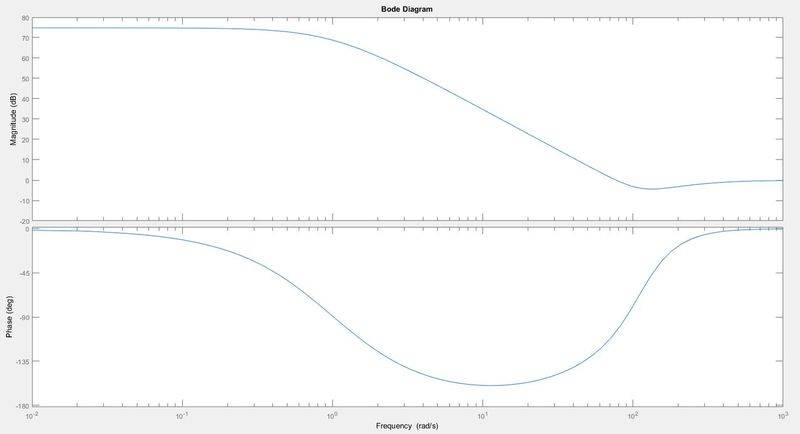Identifying type of feedback by looking at bode plot

Homework Statement

Below is the bode plot of return difference, 1+C(S)P(S) in a feedback system. Identify the frequencies where the feedback is large, negative, positive and negligible.

$$P(S) = \frac{100}{s^2+2s+1}$$

$$C(S) = \frac{220(s+50)}{s+200}$$Homework Equations

Bode Plot, Feedback

The Attempt at a Solution

Is there a way of identifying the frequencies where the feedback is large, negative, positive and negligible just by looking at the bode plot?

So the positive feedback would be where we start gaining phase & magnitude, so it'd be approximately w = 10^2.

Negligible gives you a gain of 1, so the frequency would be w = 5. Is my reasoning right?

Also what characterizes a negative and large feedback?